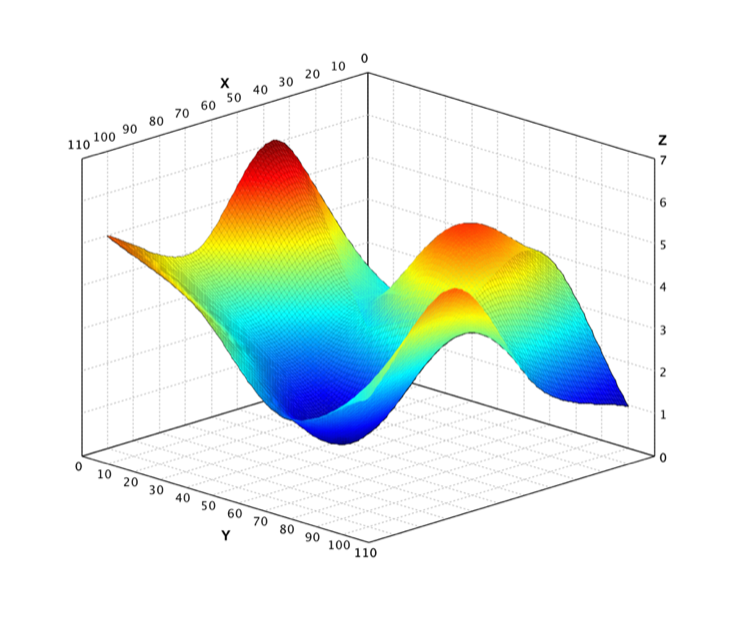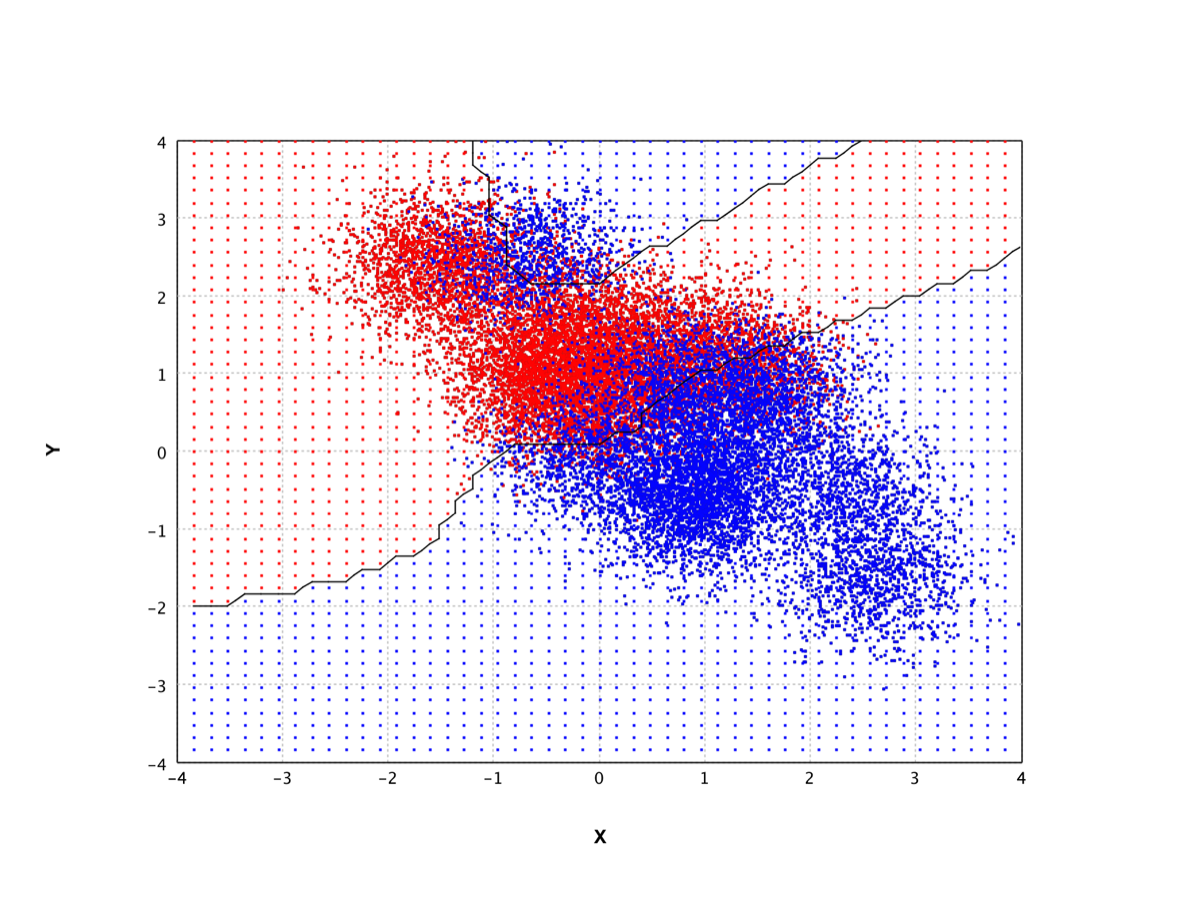Smile is a fast and comprehensive machine learning engine.

## Speed

With advanced data structures and algorithms, Smile delivers the state-of-art performance.

Compared to this third-party benchmark, Smile outperforms R, Python, Spark, H2O, xgboost significantly. Smile is a couple of times faster than the closest competitor. The memory usage is also very efficient. If we can train advanced machine learning models on a PC, why buy a cluster?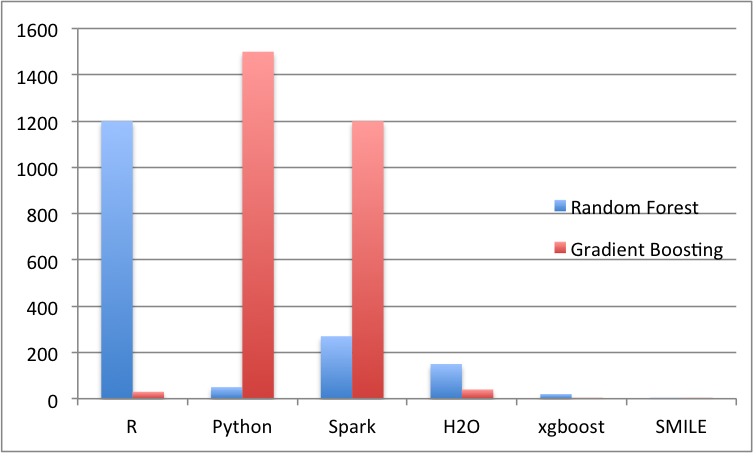Running Time (s)

## Ease of Use

Write applications quickly in Java, Scala, or any JVM languages. Data scientists and developers can speak the same language now!

Smile provides hundreds advanced algorithms with clean interface. Scala API also offers high-level operators that make it easy to build machine learning apps. And you can use it interactively from the shell, embedded in Scala.

``````

val rf = randomForest("class" ~, iris)

println(s"OOB error = \${rf.error}")
``````
Random Forest
``````
RandomForest rf = RandomForest.fit(Formula.lhs("class"), iris);
System.out.format("OOB error = %.2f%n", rf.error());
``````
Random Forest
``````
val rf = randomForest(Formula.lhs("class"), iris)
println("OOB error = \${rf.error()}")

``````
Random Forest
``````
"data/weka/iris.arff")
model (random-forest
(Formula/lhs "class") iris)]
(.error model))
``````
Random Forest

## Comprehensive

The most complete machine learning engine. Smile covers every aspect of machine learning.

Classification, regression, clustering, association rule mining, feature selection, manifold learning, multidimensional scaling, genetic algorithm, missing value imputation, efficient nearest neighbor search, etc. See the sidebar for a list of available algorithms.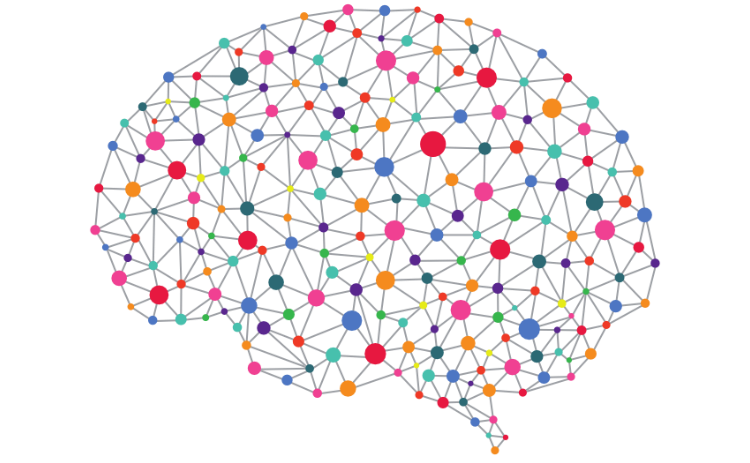## Natural Language Processing

Understanding human language, and the intent behind our words.

Tokenizers, stemming, word2vec, phrase detection, part-of-speech tagging, keyword extraction, named entity recognition, sentiment analysis, relevance ranking, taxomony.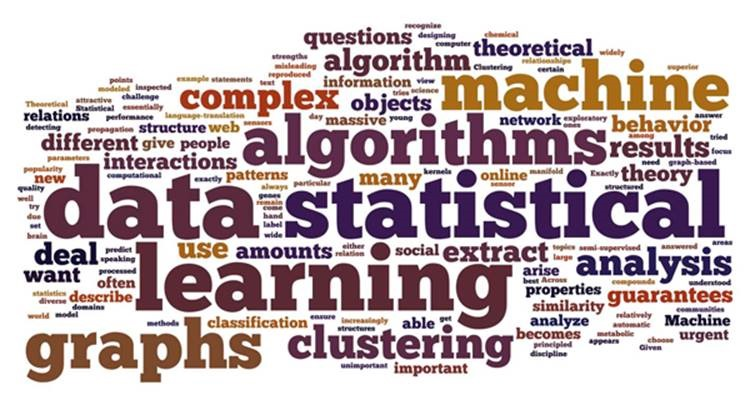## Mathematics and Statistics

Hidden gems in Smile.

From special functions, linear algebra, to random number generators, statistical distributions and hypothesis tests, Smile provides an advanced numerical computing environment. In additions, graph, wavlets, and a variety of interpolation algorithms are implemented. Smile even includes a computer algerbra system.

``````
val a = randn(3, 3)
val x = c(1.0, 2.0, 3.0)
a \ x
inv(a) %*% a
``````
Linear Algebra
``````
val bins1 = Array(8, 13, 16, 10, 3)
val bins2 = Array(4,  9, 14, 16, 7)

chisqtest2(bins1, bins2)
``````
Statistics
``````
val x = Var("x")
val y = Var("y")
val e = x**2 + y**3 + x**2 * cot(y**3)
println(e.d(x))
``````
Computer Algebra System

## Data Visualization

Interactive 2D/3D math plot.

Scatter plot, line plot, staircase plot, bar plot, box plot, heatmap, hexmap, histogram, qq plot, surface, grid, contour, dendrogram, sparse matrix visualization, wireframe, etc. Smile also supports declarative data visualization that compiles to Vega-Lite.